Cheng, L., Zhao, P., Di, Y., Dai, J., Wang, Z., Guo, X., and Wang, W. (2022). "Predictive model of the modulus of elasticity in static bending (MOE) of larch wood based on gray relation analysis (GRA) and gene expression programming (GEP)," BioResources 17(1), 445-459.

#### Abstract

To accurately evaluate the modulus of elasticity in static bending (MOE) of wooden components in ancient timberwork buildings under the “minimum intervention principle,” the nondestructive testing of physical and mechanical properties were conducted on larch. Using moisture content (MC), density (ρ), the stress wave propagation velocity ( ), the modulus of elasticity in dynamic bending (Ed), the rotational resistance value of the drilling needle (fdrill), and the resistance value of the feeding needle (ffeed) as the main parameters, the correlation between several parameters and MOE was firstly calculated using the Gray Relation Analysis (GRA) and ranked according to the strength of the correlation. Six combinations were selected according to the ranking, and the Gene Expression Programming algorithm (GEP) was used to build models for predicting MOE. The results showed that the correlation between several parameters and MOE was good (between 0.5 and 0.8), and the prediction model established with combination 6 was the best, which indicated that the prediction model established based on GRA-GEP algorithm had a certain feasibility and effectiveness, and the combined effect of the six parameters to evaluate the MOE of wooden components of ancient buildings was better in the field inspection.

Predictive Model of the Modulus of Elasticity in Static Bending (MOE) of Larch Wood Based on Gray Relation Analysis (GRA) and Gene Expression Programming (GEP)

Liting Cheng,a,c Peng Zhao,a,c Yajing Di,a,c Jian Dai,b Ziyi Wang,b Xiaodong Guo,b and Wei Wang b,*

To accurately evaluate the modulus of elasticity in static bending (MOE) of wooden components in ancient timberwork buildings under the “minimum intervention principle,” the nondestructive testing of physical and mechanical properties were conducted on larch. Using moisture content (MC), density (ρ), the stress wave propagation velocity (), the modulus of elasticity in dynamic bending (Ed), the rotational resistance value of the drilling needle (fdrill), and the resistance value of the feeding needle (ffeed) as the main parameters, the correlation between several parameters and MOE was firstly calculated using the Gray Relation Analysis (GRA) and ranked according to the strength of the correlation. Six combinations were selected according to the ranking, and the Gene Expression Programming algorithm (GEP) was used to build models for predicting MOE. The results showed that the correlation between several parameters and MOE was good (between 0.5 and 0.8), and the prediction model established with combination 6 was the best, which indicated that the prediction model established based on GRA-GEP algorithm had a certain feasibility and effectiveness, and the combined effect of the six parameters to evaluate the MOE of wooden components of ancient buildings was better in the field inspection.

DOI: 10.15376/biores.17.1.445-459

Keywords: Modulus of elasticity in static bending (MOE); Modulus of elasticity in dynamic bending (Ed); Gray relation analysis (GRA); Gene expression programming (GEP); Prediction model

Contact information: a: The Palace Museum, Beijing 100009, China; b: Faculty of Architecture, Civil and Transportation Engineering, Beijing University of Technology, Beijing 100124, China; c: Key Scientific Research Base of Study and Conservation of Guanshi Architecture in Ming and Qing Dynasty, State Administration for Cultural Heritage;

* Corresponding authors: chenglting@126.co; ieeww@bjut.edu.cn

INTRODUCTION

Wood is the main building material of ancient timberwork buildings, so it plays an important supporting role in the whole building system (Ni et al. 2019). The effective, rapid, and accurate detection of wood properties in the field without destroying the material itself and the original structure of ancient buildings has become one of the important objectives of wood research in the context of preventive conservation of ancient buildings (Li 2020). Among the wood properties, the modulus of elasticity (MOE) is one of the most important indicators, reflecting the wood’s reversible changes in dimensions when subjected to specified loads (Tian et al. 2017; Cavalheiro et al. 2018). Therefore, accurate prediction of the MOE of wood is of great practical importance. The traditional method of elastic modulus testing is carried out by mechanical experiments, but it is destructive and not suitable for ancient buildings. With the importance of assessing the timber properties of wooden components of ancient buildings and the special characteristics of testing methods, nondestructive testing (NDT) techniques have been widely applied to the field of wood timber properties in recent years (Biechele et al. 2011; Ming et al. 2013). The main NDT methods applied to the determination of wood’s elastic modulus are the ultrasonic method (Chan et al. 2011), the stress wave method (Menezzi et al. 2014), the SilviScan determination method (Dahlen et al. 2018), and the microdrill resistance meter detection method (Sun et al. 2012). These techniques have been applied extensively in the detection of wood property indicators (Haseli et al. 2020).

Based on the relevant research results on wood timber properties in recent years, there is a correlation between the MOE of wood and the NDT index. For example, Zhang, Zhu, et al. (2011) established the correlation analysis of the stress wave-drag modulus and the mechanical index. Yang et al. (2015) used NDT results to predict the MOE determined using ASTM tests with linear regression R2 values ranging from 0.77 to 0.86. Chen and Guo (2017) evaluated the mechanical performance of fir ancient wood based on NDT and reliability analysis; they found that there was a significant linear correlation between the resistance values of specimens and the MOE and the modulus of elasticity in dynamic bending (Ed). The prediction of the MOE can be carried out by nondestructive testing technology, which will provide important significance for the accurate evaluation of the load-bearing capacity of wooden elements of ancient buildings under the “principle of minimum intervention” and preventive conservation.

Gray relation analysis (GRA) is a multifactorial statistical analysis. It is based on the sample data of each factor and uses the gray correlation degree to describe the strength, magnitude, and order of the relationship between the factors (Zhang et al. 2011). Generally, if the correlation degree is greater than or equal to 0.8, it is considered to have good correlation. If the correlation degree is between 0.5 and 0.8, it is considered to have correlation. If the correlation degree is less than 0.5, it is basically irrelevant (Chen et al. 2016). Li et al. (2020) discriminated the residual damage area of the specimens under the stress wave method, applied the GRA method, combined with the stepwise discrimination method to establish the model, and finally obtained a high discrimination accuracy.

Gene expression programming (GEP) is a newly developed evolutionary method. It is developed from genetic algorithm (GAS) and genetic programming (GP). Gene expression programming overcomes the complex functions in the GAS system and the difficulty of genetic operations in the GP system. Gene expression programming also has stronger function discovery ability and higher search efficiency, which can realize data mining in multidimensional space. It is currently the most effective analysis method for complex nonlinear problems (Ferreira 2001). Currently, GEP has been studied in relation to the dynamic modulus of asphalt mixes (Yan et al. 2015), concrete bond strength (Zhang and Xue 2021), subway civil engineering cost estimation (Wang and Lu 2019), and abrasive jet cutting depth (Wang et al. 2015). In addition, more desirable prediction models have been established to improve the prediction accuracy.

Therefore, in order to quickly and accurately predict the static bending (MOE) of ancient wood structures, gray relation analysis (GRA) and gene expression programming (GEP) were introduced to establish a predictive model of the modulus of elasticity in static bending (MOE) of larch wood based on nondestructive testing. To be specific, this study took larch as the research object. Firstly, the moisture content (MC), density (ρ), stress wave propagation velocity (), the modulus of elasticity in dynamic bending (Ed), the rotational resistance value of the drilling needle (fdrill), and the resistance value of the feeding needle (ffeed) were obtained by means of stress wave detection method, microdrill resistance meter detection method, and standard experiments. Secondly, the correlation degree between these six parameters and MOE of wood were calculated by using GRA, respectively. Six combinations were selected according to the strength of correlation degree. Finally, GEP was used to establish the predictive model of the modulus of elasticity in static bending (MOE) of larch wood. The prediction results show that this method can predict quickly and accurately the MOE of ancient wooden structure, which provides a new method for the safety evaluation of ancient wooden building.

EXPERIMENTAL

Materials

The experimental material was larch, whose scientific name is Larix gmelinii. The experimental material was purchased from Qing Dongling Wood Factory in Zunhua City, Hebei Province. The diameters of the base and the top were 50 cm and 40 cm, respectively. The height was 400 cm. In order to facilitate the production of the specimen, the wood was divided into 8 equal sections which included sections A, B, C, D, E, F, G, and H. The length of each section was 50 cm. In addition, in order to ensure that the test piece could cover the whole wood, the specimens were selected from 10 annual rings of depth in each cross-section, as shown in Fig. 1.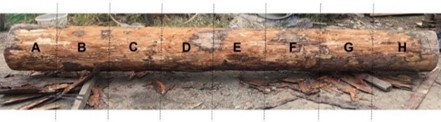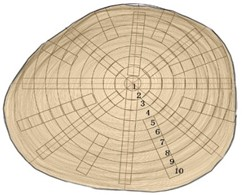Fig. 1. Specimen sawing pattern

According to the determination requirements of MOE in “Method of sample logs sawing and test specimens selection for physical and mechanical tests of wood (GB/T 1929-2009)”, the standard specimen size should be 2 cm × 2 cm × 36 cm (transverse × radial × longitudinal). In order to ensure that the moisture content (MC), density (ρ), stress wave propagation velocity (), the rotational resistance value of the drilling needle (fdrill), and the resistance value of the feeding needle (ffeed) were obtained from the same specimen, we finally selected a specimen with a size of 2 cm × 2 cm × 45 cm (transverse × radial × longitudinal). Each specimen was marked with moisture content testing area, density testing area, stress wave testing area, impedance meter testing area, and MOE testing area, as shown in Fig. 2.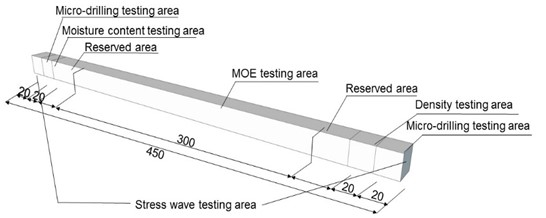Fig. 2. Experimental specimen partition (unit: mm)

Methods and Steps

The size of the specimens was measured with vernier calipers (Guilin Guanglu Measuring Instrument Co., Guilin, China), so that the volume (V) of the sample could be calculated. The mass (m) of the specimens was measured with a precision electronic balance (Shanghai Lichen Electronic Technology Co., Shanghai, China). Then, the density (ρ) of the sample could be calculated according to Eq. 1,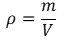(1)

The propagation time of the stress wave in the wood was measured with a stress wave tester (FAKOPP Enterprise Bt., Ágfalva, Hungary). Two transducers were coupled in the specimens’ ends, and measurements were made along the direction parallel to the fibers of the specimens. The angle between the two transducers and the length of the specimens was not less than 45°, and the distance between the two measurement points was measured. During the measurement, the reading of the propagation time of the first tap was invalid. From the second measurement, the average value of the propagation time obtained by continuously measuring three times was used as the final test result.

The micro-drilling resistance instrument 200/5000 (the feed speed was 200 cm/min, needle speed was 5000 r/min) was used to measure both ends of the specimens, and the resistance value of the material inside the wood was tested. A transducer was drilled into the surface of the processed specimen at a uniform speed and perpendicular to the direction of the annual ring, and the test data was imported into the computer to calculate the resistance value. The resistance value included the fdrill and ffeed values.

In accordance with the location shown in Fig. 2, a small specimen of 3 cm × 2 × cm × 2 cm (longitudinal, transverse, and radial) was sawed at one end of the specimen, and the MC of the specimen was measured according to “Method for determination of the moisture content of wood (GB/T1931-2009)”. According to “Method for determination of the modulus of elasticity in static bending of wood (GB/T 1936.2-2009)”, the universal mechanical testing machine produced by Jinan Rima Machinery Equipment Co., Ltd. was used to measure the MOE of wood.

The theoretical Ed of wood can be calculated according to Eq. 2,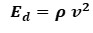(2)

(Ross et al. 2004).

So the theoretical Ed of wood can be calculated by substituting Eq. 1 into Eq. 2,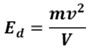(3)

Gray Relation Analysis

In order to determine the degree of influence of the parameters on the MOE, the GRA algorithm was used for the analysis, and its calculation steps can be seen below.

(1) Determine the analysis sequence. In this paper, the MOE was used as the parent sequence Y=Y(k), MC, ρ, , Ed, fdrill, and ffeed. Six parameters were the subsequence, Xi=Xi(k), where i=1, 2, 3, 4, 5, 6；k=1, 2, …152.

(2) Dimensionless processing of sequences (normalization). The commonly used methods include the mean value method and the initialization method. This paper adopted initialization method, which can be calculated according to Eq. 4,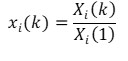(4)

where i=1, 2, …n.

(3) The difference series, the maximum difference, and the minimum difference can be obtained by Eq. 5, Eq. 6 and Eq. 7, respectively.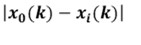(5)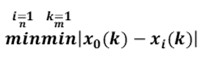(6)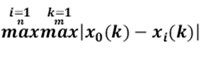(7)

where, k=1, 2, m; i=1, 2, …n.

(4) The number of correlation coefficients can be calculated according to Eq. 8,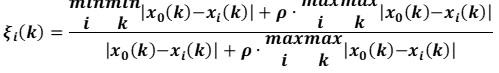(8)

where, the resolution factor was ρ equal to 0.5.

(5) The correlation can be calculated according to Eq. 9,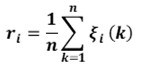(9)

(6) Sorted by relevance. The correlation between the comparison sequence and the reference sequence was sorted from largest to smallest.

RESULTS AND DISCUSSION

Establish the MOE Prediction Model based on GRA-GEP

Experimental data analysis and processing

The MC, ρ, , Ed, fdrill, and ffeed values were obtained according to the stress wave detection method, microdrill resistance meter detection method, and standard experiments. There were 152 groups of experimental data. Statistical parameters are shown in Table 1.

Table 1. Statistical Parameters of Experimental Data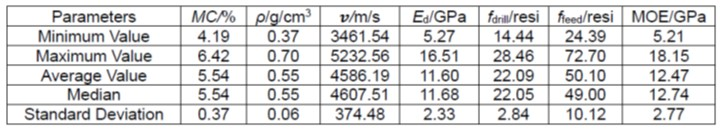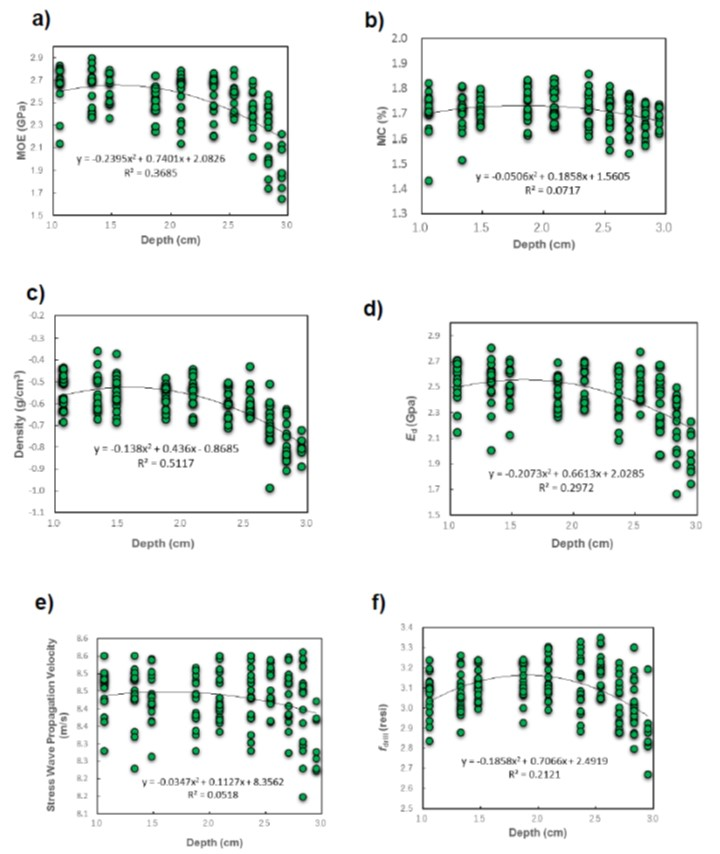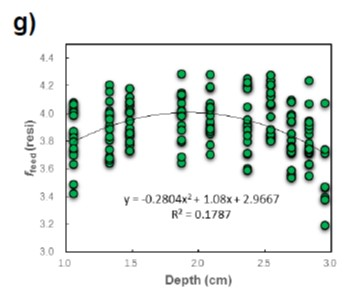Fig. 3. The trends of the a) MOE, b) MC, c) ρ, d) Ed, e) , f) fdrill, and g) ffeed values with the annual rotation depth

In order to reduce the absolute value of the data and facilitate the calculation, the logarithms of the original data were treated preliminarily. Figure 3 shows that the variation trend of MOE, MC, ρ, , Ed, fdrill and ffeed at different ring depths. It can be seen from Fig. 3 that the other six parameters except all showed certain regularity with different tree ring depth.

Similarly, Fig. 4 shows that the variation trend between six parameters and MOE. Formulae fitting was carried out on these variation trend graphs. The fitting results are listed in Table 2. The fitting results showed that MOE had certain correlation with other 5 parameters except MC, among which MOE has relatively good correlation with Ed and ρ.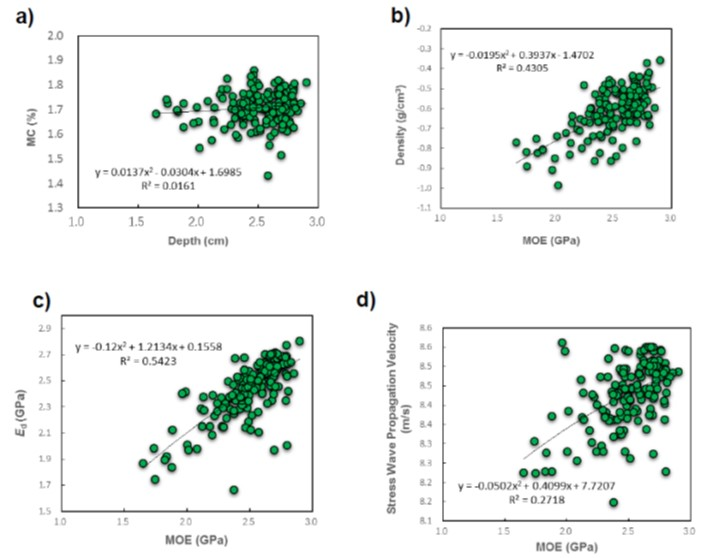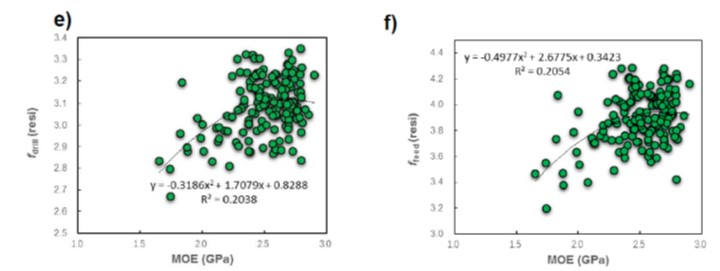Fig. 4. Fitting relationship between the MOE and the a) MC, b) ρ, c) Ed, d) , e) fdrill, and f) ffeed values

From the experimental procedure and results, the six parameters have some correlation. In order to determine the degree of influence of the six parameters on the MOE, the GRA was used for analyzing the 152 data. The correlation ranking of the MOE is shown in Table 3. That is, the correlations of the six parameters with the MOE were ranked from strongest to weakest as follows: Ed ffeed ρfdrill ＞ ＞ MC.

Table 2. Relationship Between the MOE and MC, ρ, , Ed, fdrill, and ffeed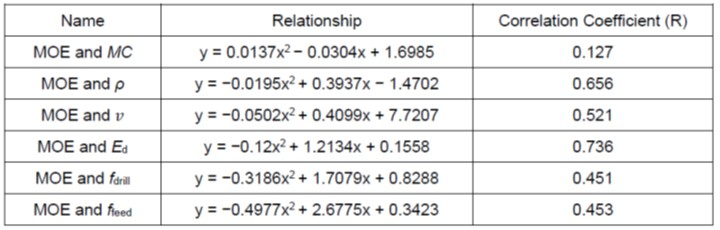Table 3. Relevance Ranking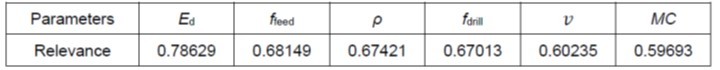Establishing the GRA-GEP models

The GeneXproTools 5.0 software (Bristol, UK) was used to establish a more accurate prediction model for the MOE of the larch wood. It was derived by varying various parameters such as gene number, chromosome number and linkage function, and selecting the number of evolutionary generations. The optimal parameters obtained from the tests are shown in Table 4.

Table 4. Parameter for the GEP Models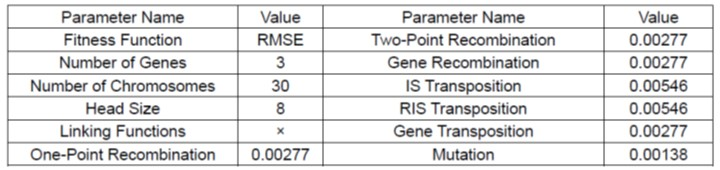The set of functions chosen was F= {+, -, *, /, exp(x), ln(x), x2, 1/x, x1/3}. The set of variables was T= {d0, d1, d2, d3, d4, d5}, where d0~d5 were MC, ρ, V, Ed, fdrill, and ffeed, and the output value was the MOE. The flow chart of the GEP algorithm is shown in Fig. 5.

Six combinations were selected for the GEP models according to the ranking of the correlations. The comparison results are shown in Table 5.

Table 5. Combination Approach and Model Results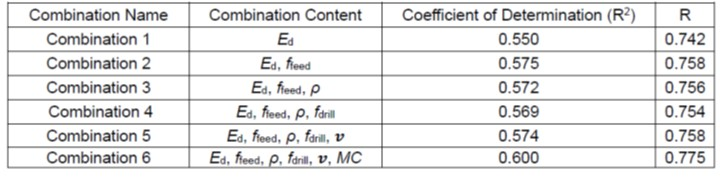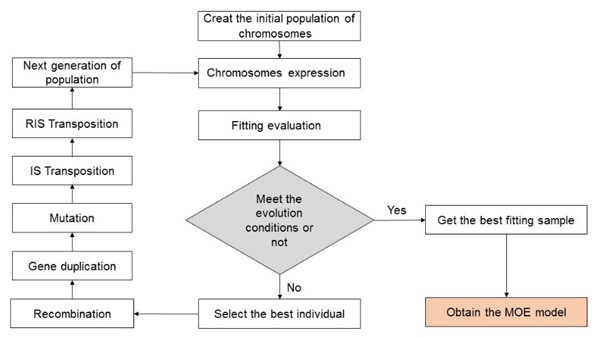Fig. 5. The GEP algorithm flow chart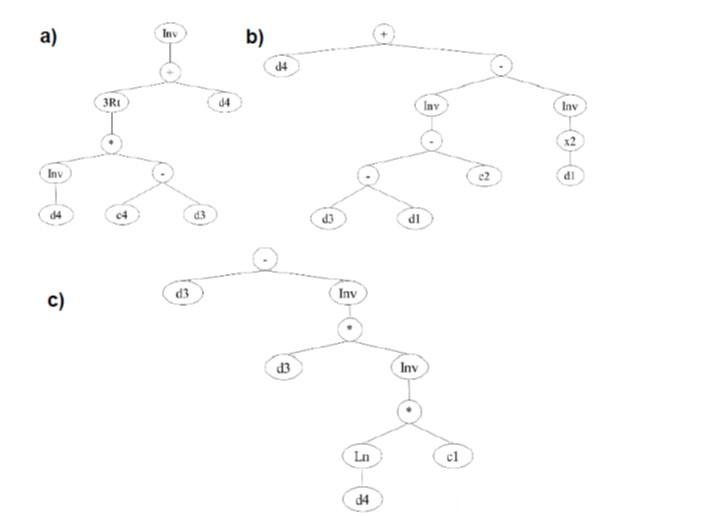Fig. 6. The expression tree for a) Sub-ET 1, b) Sub-ET 2, and c) Sub-ET 3 of combination 6

From the R2 and R values for the six combinations, the optimal prediction model was obtained for combination 6. That is, all six parameters had some effect on the prediction of the MOE. The prediction model was obtained using the GeneXproTools 5.0 software as follows:

G1C4 = −4.55449079866939;

G2C2 = 6.51335825678274;

G3C1 = −8.75511671485119;

y = 0.0;

y = (1.0/((gep3Rt(((1.0/(d(5)))*(G1C4−d(4))))+d(5))));

y = y * (d(5)+((1.0/(((d(4)−d(2))−G2C2)))−(1.0/((d(2)^2)))));

y = y * (d(4)−(1.0/((d(4)*(1.0/((reallog(d(5))*G3C1)))))));

result = y;

function result = gep3Rt(x)

if (x < 0.0),

result = −((-x)^(1.0/3.0));

else

result = x^(1.0/3.0);

end

The expression tree for the corresponding combination 6 is shown in Fig. 6.

Analysis of the GRA-GEP Models

The coefficient of determination (R2), Mean Squared Error (MSE), Residual Standard Error (RSE), Root Mean Squared Error (RMSE), and Mean Absolute Error (MAE) were used to test the feasibility and accuracy of the prediction model. The formulas for the R2, the MSE, the RSE, the RMSE, and the MAE values can be seen in Eqs. 10, 11, 12, 13, and 14, respectively,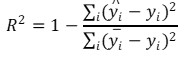(10)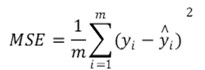(11)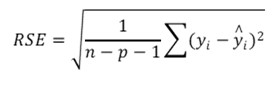(12)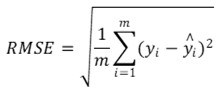(13)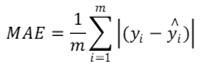(14)

where,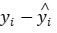is the difference between the true value and the predicted value, n-p-1 is the degree of freedom, and p is the number of features.

The results of the evaluation index calculation for the six combinations are shown in Table 6. The results in Table 6 showed that combination 6 was superior to the other combinations in all the evaluation indicators. This indicated that combination 6 was better again.

Table 6. Evaluation Metrics for the Six Combinations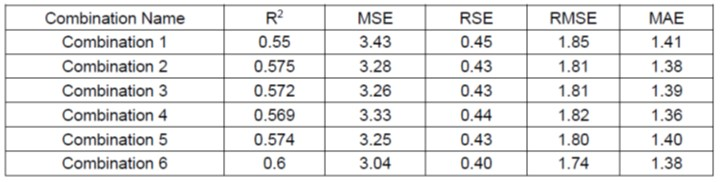To select the optimal prediction model, the above five indicators were normalized to obtain the expression for the comprehensive evaluation accuracy, as seen in Eq. 15 (Wang et al. 2020),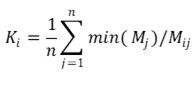(15)

where Ki is the comprehensive evaluation of the i-th combination, i=1, 2,…6, Mij is the j-th index of the i-th combination, j=1, 2,…, and min (Mj) is the minimum value among the j-th index of the six combinations. A higher Ki value indicates a better combination.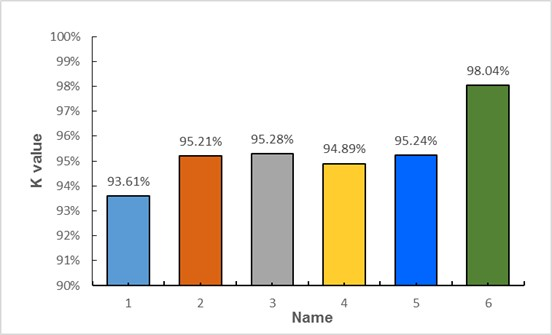Fig. 7. Comprehensive evaluation accuracy of the six combinations

Figure 7 shows that the evaluation accuracy value of combination 6 was significantly better than the other five combinations. This indicated that the prediction model built using the six parameters can improve the prediction accuracy of the MOE. The K-values of the six combination prediction models were compared and analyzed, and the K-value of the combination 6 prediction model was 98.04%, which was the largest among all the combination prediction models. In summary, the prediction model established by combination 6 was the optimal model, followed by combinations 3, 5, 2, 4, and 1. The prediction model indicated that the data of the six parameters should be integrated to assess the MOE values of wooden elements of ancient buildings in the field of testing.

LIMITATIONS

This study has certain limitations. Only one test material, which is larch wood, was used to predict the modulus of elasticity in static bending. Since different tree species have different wood properties, more tree species samples (such as fir, cypress, nanmu, etc.) need to be considered in the follow-up research. In addition, because the material properties of wood are also affected by damage conditions (such as decay, cracks, insect attacks, etc.), the influence of these factors need to be considered in the follow-up research. In this way, a more complete database can be established to enhance the applicability of the model.

CONCLUSIONS

1. The Gray Relation Analysis – Genetic Expression Programming (GRA-GEP) algorithm was introduced to predict the modulus of elasticity (MOE) of wood, and the experimental demonstration revealed that the constructed prediction model was highly accurate, feasible, and effective.
2. Through gray correlation analysis, it was found that the correlation between the six parameters and the MOE was ranked from strongest to weakest as follows: Ed > ffeed > ρ > fdrill > > MC.
3. In comparing the prediction models of six combinations, calculating the statistical index values, and normalizing them to calculate the evaluation accuracy, the comparison results showed that the prediction accuracy of combination 6 was optimal. The prediction model indicated that the six parameters should be integrated to evaluate the MOE values of wooden elements of ancient buildings in the field of inspection.

ACKNOWLEDGMENTS

The authors are grateful for the support of the National Key R&D Program (2019YFC1520902 and 2020YFC1522402) and the Beijing Municipal Commission of Education-Municipal Natural Science Joint Foundation (Grant No. KZ202010005012). Also thanks to a special grant from the Forbidden City Cultural Heritage Conservation Foundation and the Vanke Foundation.

REFERENCES CITED

Biechele, T., Chui, Y. H., and Gong, M. (2011). “Comparison of NDE techniques for assessing mechanical properties of unjointed and finger-jointed lumber,” Holzforschung 65(3), 397-401. DOI: 10.1515/hf.2011.038

Cavalheiro, R. S., de Almeida, D. H., de Almeida, T. H., Christoforo, A. L., and Lahr, F. A. R. (2018). “Estimation of modulus of elasticity in static bending of wood in structural dimensions as a function of longitudinal vibration and density,” Current Journal of Applied Science and Technology 26(1), 1-8. DOI: 10.9734/CJAST/2018/39531

Chan, J. M., Walker, J. C., and Raymond, C. A. (2011). “Effects of moisture content and temperature on acoustic velocity and dynamic MOE of radiata pine sapwood boards,” Wood Science and Technology 45(4), 609-626. DOI: 10.1007/s00226-010-0350-6

Chen, Q., Zhang, Q., Chen, X., Xiao, C., and Jiang, Q. (2016). “Prediction of blasting-vibration-peak-speed based on GRA-GEP,” Journal of Central South University (science and technology) 47(7), 2441-2447. DOI: 10.11817/j.issn.1672-7207.2016.07.035

Chen, Y., and Guo, W. (2017). “Nondestructive evaluation and reliability analysis for determining the mechanical properties of old wood of ancient timber structure,” BioResources 12(2), 2310-2325. DOI: 10.15376/biores.12.2.2310-2325

Dahlen, J., Antony, F., Schimleck, L. R., and Daniels, R. F. (2018). “Relationships between static mechanical properties and SilviScan measured wood properties in loblolly pine,” Forest Products Journal 68(1), 37-42. DOI: 10.13073/FPJ-D-15-00044

Ferreira, C. (2001). “Gene expression programming: A new adaptive algorithm for solving problems,” Complex Systems 13(2), 87-129. DOI: 10.1007/3-540-32849-1

GB/T 1929-2009. “Method of sample logs sawing and test specimens selection for physical and mechanical tests of wood,” Standardization Administration of China, Beijing, China.

GB/T1931-2009. “Method for determination of the moisture content of wood,” Standardization Administration of China, Beijing, China.

GB/T 1936.2-2009. “Method for determination of the modulus of elasticity in static bending of wood,” Standardization Administration of China, Beijing, China.

Haseli, M., Layeghi, M., and Hosseinabadi, H. Z. (2020). “Evaluation of modulus of elasticity of date palm sandwich panels using ultrasonic wave velocity and experimental models,” Measurement 149, 107016. DOI: 10.1016/j.measurement.2019.107016

Li, X. (2015). Key Technology Research on Material Performance and Damage Detection for Wooden Components of Ancient Chinese Building, Ph.D. Dissertation, Beijing University of Technology, Beijing, China.

Li, X., Qian, W., Cheng, L., and Chang, L. (2020). “A coupling model based on grey relational analysis and stepwise discriminant analysis for wood defect area identification by stress wave,” BioResources 15(1), 1171-1186. DOI: 10.15376/biores.15.1.1171-1186

Menezzi, C. H. S. D., Amorim, M. R. S., Costa, M. A., and Garcez, L. R. O. (2014). “Evaluation of thermally modified wood by means of stress wave and ultrasound nondestructive methods,” Materials Science 20(1), 61-66. DOI: 10.5755/j01.ms.20.1.3341

Ming, C.-M., Lin C.-H., and Yang T.-H. (2013). “Application of nondestructive methods to evaluate mechanical properties of 32-year-old Taiwan incense cedar (Calocedrus formosana) wood,” BioResources 8(1), 688-700. DOI: 10.15376/biores.8.1.688-700

Ni, Y., Tang, G., Zhang, Z., Wang, C. (2019). “Study on the protection and repair methods of ancient wooden structures: Take the Diaodongge in Jingxian as an example,” Value Engineering 38(23), 238-240. DOI: 10.14018/j.cnki.cn13-1085/n.2019.23.100

Ross, R. J., Brashaw, B. K., Wang, X., White, R. W., and Pellerin, R. F. (2004). Wood and Timber Condition Assessment Manual, USDA Forest Products Society, Madison, WI, USA.

Sun, Y., Zhu, L., Wang, X., Yan, H., and Liao, C. (2012). “Research on rapid detection of larch wood modulus of elasticity based on micro-drilling resistance,” Hubei Agricultural Sciences 51(11), 2348-2350. DOI: 10.14088/j.cnki.issn0439-8114.2012.11.014

Tian, Z., Wang, Z., Wang, J., Zhang, Z., Ge, B., Le, Y., and Ren, H. (2017). “Classification in modulus of elasticity and mechanical properties of larch lumber in Mohe,” Journal of Northwest Forestry University 32(3), 211-215. DOI: 10.3969/j.issn.1001-7461.2017.03.39

Wang, J., and Lu, Y. (2019). “Cost estimation of metro tunnel civil engineering based on genetic expression programming,” Journal of Changsha University of Science and Technology 16(3), 17-24. DOI: CNKI:SUN:HNQG.0.2019-03-003

Wang, R., Zhong, G., Zhou W., Li, L., Yang, H., and Wang F. (2015). “A model for predicting cutting depth of casing using AWJ technique based on gene expression programming algorithm,” Journal of China University of Petroleum 39(1), 60-65. DOI: 10.3969/j.issn.1673-5005.2015.01.008

Wang, Z., Wang, W., Ma, D., Guo, X., Huan, J., and Cheng, L. (2020). “Coupling model of fuzzy soft set and Bayesian method to forecast internal defects of ancient wooden structures based on nondestructive test,” BioResources 15(1), 1134-1153. DOI: 10.15376/biores.15.1.1134-1153

Yang, B. Z., Seale, R. D., Shmulsky, R., Dahlen, J., and Wang X. (2015). “Comparison of nondestructive testing methods for evaluating no. 2 southern pine lumber: Part A, modulus of elasticity,” Wood and Fiber Science: Journal of the Society of Wood Science and Technology 47(4), 375-384.

Yan, K., Liu, P., and Wang, X. (2015). “Prediction of dynamic modulus of asphalt mixture based on gene expression programming algorithm,” Journal of Building Materials 18(6), 1106-1110. DOI: 10.3969/j.issn.1007-9629.2015.06.034

Zhang, H., Zhu, L., Sun, Y., and Wang, X. (2011). “Determining main mechanical properties of ancient architectural timber,” Journal of Beijing Forestry University 33(5), 126-129. DOI: 10.13332/j.1000-1522.2011.05.007

Zhang, Q.-l., Wang, Y.-l., and Cao X.-g. (2011). “Backfill strength prediction based on the grey-neural network,” Industrial Minerals & Processing 40(12), 26-28, 37. DOI: 10.3969/j.issn.1008-7524.2011.12.008

Zhang, R., and Xue, X. (2021). “Bond strength prediction model of the near-surface-mounted fiber-reinforced polymer concrete based on gene expression programming,” Advanced Engineering Sciences 53(2), 118-124. DOI: 10.15961/j.jsuese.202000405

Article submitted: July 31, 2021; Peer review completed: September 24, 2021; Revised version received: October 24, 2021; Accepted: November 19, 2021; Published: November 23, 2021.

DOI: 10.15376/biores.17.1.445-459